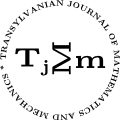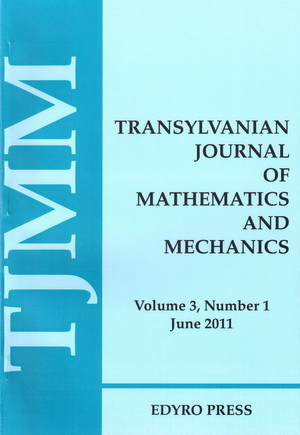ISSN: 2067-239X
ISSN(on-line): 2067-239X

Indexed in:
Mathematical Reviews
Zentralblatt MATH
EBSCO

Front cover# Volume 1 (2009), Number 1-2

## Green’s Functions in Mathematical Physics

### Author(s): WILHELM KECS

Abstract: The determination of Green functions for some operators allows the effective writing of solutions to some boundary problems of mathematical physics.

## Distributions from ${D}^{\prime }\left({ℝ}^{n}\right)$ Representable only with Respect to the Polar Coordinate $r\in ℝ$

### Author(s): WILHELM W. KECS

Abstract: The notion of distribution from ${D}^{\prime }\left({ℝ}^{n}\right)$ representable only with respect to the polar coordinate $r\in ℝ$ is defined. Some of its properties are proved. It is shown that the distributions $\delta x$, $\Delta \delta \left(x\right)\in {D}^{\prime }\left({ℝ}^{n}\right)$ and others are representable with respect to $r\in ℝ$ and their expressions are given.

## A Nonlinear Fredholm Integral Equation

### Author(s): MARIA DOBRIȚOIU

Abstract: This paper contains several results of existence, existence and uniqueness and continuous data dependence of the solution of a nonlinear Fredholm integral equation with modified argument, which appears in the 70', in some problems from turbo-reactors industry. To obtain these results the Contraction Principle, the Schauder's theorem and the General data dependence theorem are useful.

Abstract: We study in this paper the Gauss-Christoffel with fixed nodes quadrature formula with their principal's properties, looking up to construct a new Gauss-Laguerre-Radau quadrature formula with one fixed nodes, corresponding to the Laguerre orthogonal polynomials. We gave too an application to this formula.

## Variation of Curves Length Reported to Cone Metric in Lorentz Manifold

### Author(s): SORIN NOAGHI

Abstract: On Lorentz manifold $\left(M,g\right)$ we consider a timelike, parallel and unitary vector field $Z$ with its help we define the $Z$-length of curves and we obtain their first and the second variation.

## Linking Devices for Wide Cables to Balance Winding Installations

### Author(s): DUMITRESCU IOSIF, JULA DUMITRU, AND ITU VILHELM

Abstract: Linking devices for wide balancing cables connect between extraction vessel and wide cable to compensate for vertically transported masses. Such a device is made up of a series of elements of resistance that make connection between the bottom of the extraction vessel and the exterior core, whose operational width is determined by the width of the wide cable and a series of fixing clamps of the cable end wrapped around the core. In the paper is present the numerical analysis with finite elements DLCLE118 device.